# Simply-periodic function

(diff) ← Older revision | Latest revision (diff) | Newer revision → (diff)
A periodic functionof the complex variableall periodsof which are integer multiples of a single unique fundamental, or primitive, period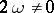, i.e.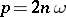(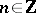). For example, the exponential function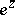is an entire simply-periodic function with fundamental period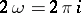, and the trigonometric functions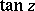and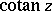are meromorphic simply-periodic functions with fundamental period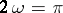.
More generally, a simply-periodic function on a linear spaceis a periodic function whose periods are integer multiples of some basic period. A non-constant continuous periodic function of a real variable is necessarily simply-periodic.